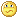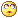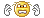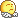#www.vustudents.ning.com

We non-commercial site working hard since 2009 to facilitate learning Read More. We can't keep up without your support. Donate.

# CS304- Object Oriented Programming.Assignment No. 03 SEMESTER Spring 2013.due date : 20/05/2013

Assignment No. 03
SEMESTER Spring 2013
CS304- Object Oriented Programming

Total Marks: 20

Due Date: 20/05/2013

Instructions

It should be clear that your assignment will not get any credit (zero marks) if:

• The assignment is submitted after due date.
• The submitted assignment is other than .cpp file.
• The submitted assignment does NOT open or file is corrupted.
• The assignment is copied (from other student or ditto copy from handouts or internet).

• For clarity and simplicity, you are required to Upload/Submit only .CPP file

Objective

The objective of this assignment is:

• To give you the idea of practical implementation of some concepts like, definition of classes, Data members, member functions, Aggregation, Composition, Constructors etc.

For any query about the assignment, contact at CS304@vu.edu.pk

GOOD LUCK

Marks: 20

Composition and Aggregation is an important relationship between objects in object oriented paradigm. Consider the following class diagram, which shows some classes and their relationship (Composition and Aggregation), while the detail of classes is given in the table.

 Class Name Attribute Name Attribute Data Type Functions Sign Name Character String -Constructor -Getters and Setters Candidate Name Character String -Default constructor() Votes_obtain Integer -Overloaded Constructor sign Sign -Getters and Setters -Count_votes() Constituency ConNo Character String -Default constructor() Candidate_name Cadidate -Overloaded Constructor -Getters and Setters Result DayOf_result Character String -Default constructor -Getters and Setters -compile_result() VB_Paper CanName Candidate -Default constructor res Result -Overloaded Constructor -Getters and Setter -Get_result()

You are required to implement this class diagram (Write complete program) in to C++ with all functions and concepts (Aggregation and composition) given in the class diagram/table.

You need to consider that there may be two or more than two candidates who can contest in the constituency.

Details of important Member functions:

compile_result() : This function will Compile (calculate) the result of the contesting candidates.

Get_result(): This function will get/show result from the Result class through the part object “res” of the VB_Paper class.

Output:

Lectures Covered:  This assignment covers Lecture #    07-15

Views: 7903

Attachments:

### Replies to This Discussion

#include <iostream>
#include <string.h>
using namespace std;
//Sign Class
class Sign
{
private:
//variable for holding sign
char* name;
public:
//Constructor
Sign()
{
name = NULL;
}
void setName(char* n)
{
if(this->name != NULL)
delete name;
name = new char[strlen(n) + 1];
strcpy(name, n);
}
char* getName()
{
return this->name;
}

};
class Candidate
{
private:
//variables for holding name, total votes and Sign of candidate
char* name;
Sign* sign;

public:
Candidate()
{
name = NULL;
sign = NULL;

}
Candidate(const char* n, int votes, Sign* sign)
{

setName(n);

setSign(sign);
}
void setName(const char* n)
{
if(this->name != NULL)
delete name;
name = new char[strlen(n) + 1];
strcpy(name, n);
//this->index = 0;
}
const char* getName()
{
return this->name;
}
//method for setting the total votes of candidate
{

}
{
}
{
}
void setSign(Sign* s)
{
this->sign = new Sign();
this->sign->setName(s->getName());
}
Sign* getSign()
{
return this->sign;
}
};
class Result
{
private:
//varible for holding winner name
char* result;
public:
Result()
{
result = NULL;
}
void setResult(const char* res)
{
if(result != NULL)
delete result;
result = new char[strlen(res) + 1];
strcpy(result, res);
}
const char* getResult()
{
return this->result;
}
//This method will get the winner based on total votes cast to candidate
void compileResult(Candidate* candidates[], int totalCandidates)
{
int currentWinner = 0, currenthigherVotes = 0;
for(int i = 0; i < totalCandidates; i++)
{
//if the previous higher votes is lesser then this candidate, then this is current higher voter candidate
{
//saving the index of winner candidate
currentWinner = i;

}
//If the current higher votes ties with this candidate flag the current higher votes to 0 or in case no vote cast
{
}
}
//if current higher votes is not 0 means we have a winner
{
this->setResult(candidates[currentWinner]->getName());
}
//else means the result is tie or no vote cast
else
{
this->setResult("No Winner");
}

}

};
class VB_Paper
{
private:
//variables for holding candidates and result
Candidate* candidate;
Result result;
public:
VB_Paper()
{
for(int i = 0; i < 2; i++)
{
candidate[i] = NULL;
}

}
VB_Paper(Candidate* can[], int totalCandidates)
{
setCandidates(can, totalCandidates);
}
Result getResult()
{
return this->result;
}

void setCandidates(Candidate* can[], int totalCandidates)
{
for(int i = 0; i < totalCandidates; i++)
{
this->candidate[i] = can[i];
}

}

//method for assigning the vote to candidate of given sign
void setVoteForSign(char* sign)
{
for(int i = 0; i < 2; i++)
{
if(!strcmp(this->candidate[i]->getSign()->getName(), sign))
{
break;
}

}
}
//method for displaying the winner and how much vote each candidate got
void GetResult()
{
//compile the result of all candidates
result.compileResult(this->candidate, 2);
cout "\nWinner is " result.getResult();
cout "\n====================================\n";
cout "\nCandidate Name \t Votes Obtained";

for (int i = 0; i < 2; i++)
{
cout "\n" candidate[i]->getName() " \t " candidate[i]->Count_Votes();
}
}
};
class Contituency
{
private:
//variables for holding contituency no, candidates and VB_Paper
char* contituencyNumber;
Candidate* candidates;
VB_Paper* paper;

public:
Contituency()
{
contituencyNumber = NULL;
for(int i = 0; i < 5; i++)
{
candidates[i] = NULL;
}
paper = NULL;
}
Contituency(char* conNo, Candidate* can[], int totalCandidates)
{
setContituencyNumber(conNo);
setCandidates(can, totalCandidates);

}
void setVB_Paper(Candidate* can[], int totalCandidates)
{
paper = new VB_Paper(can, totalCandidates);
}
void setContituencyNumber(char* conNo)
{
if(this->contituencyNumber && this->contituencyNumber != NULL)
delete contituencyNumber;
contituencyNumber = new char[strlen(conNo) + 1];
strcpy(this->contituencyNumber, conNo);
}
const char* getContituencyNumber()
{
return this->contituencyNumber;
}
void setCandidates(Candidate* can[], int totalCandidates)
{
for(int i = 0; i < totalCandidates; i++)
{
this->candidates[i] = can[i];
}
}
Candidate** getCandidates()
{
return this->candidates;
}
//Method for taking input and setting candidates
void start()
{

Candidate* can = {new Candidate(), new Candidate()};

Sign* sign1 = new Sign();
sign1->setName("Hockey");
can->setName("Anwer Abbasi");
can->setSign(sign1);

Sign* sign2 = new Sign();
sign2->setName("Ball");
can->setName("Eshan Malik");
can->setSign(sign2);

char input;
cout "\nEnter Contituency Number/Name: ";
cin >> input;

this->setContituencyNumber(input);
this->setCandidates(can, 2);
this->setVB_Paper(can, 2);

cout "\n\n=========== Contituency: "this->contituencyNumber " Election" " =============" endl;
cout "\n\nContesting Candidates are: " endl;

cout "\n====================================================";
cout "\nCandidate Name \tSign";
for (int i = 0; i < 2; i++)
{
cout "\n" candidates[i]->getName() " \t" candidates[i]->getSign()->getName();
}
char voteInput;
char* signName1 = candidates->getSign()->getName();
char* signName2 = candidates->getSign()->getName();
while(true)
{
cout "\n Do you want to cast vote:(Y/N): ";
cin >> voteInput;
if(!strcmp(voteInput, "Y"))
{

cout "\nSelect your option: " " (1 for " signName1 " and 2 for " signName2 ")" endl;
cout "\n 1. " signName1;
cout "\n 2. " signName2;
cout endl;
cin >> input;
if(!strcmp(input, "1"))
{
//assign the vote from paper to candidate have a given sign
this->paper->setVoteForSign(signName1);
}
else
{
this->paper->setVoteForSign(signName2);
}
}
else
{
cout "\n Do you want to view result (Y/N): ";
cin >> input;
if(!strcmp(input, "Y"))
{ //get the result
paper->GetResult();
}
cout "\n\n Thanks for using program.\n\n";
break;
}
}

}

};

int main()
{
//Create contituency and start flow
Contituency* con = new Contituency();
con->start();
system("pause");
return 0;
}

thnkooooooo#include <iostream>
#include <string.h>
using namespace std;

//Sign Class
class Sign
{
private:
//variable for holding sign
char* name;
public:
//Constructor
Sign()
{
name = NULL;
}
void setName(char* n)
{
if(this->name != NULL)
delete name;
name = new char[strlen(n) + 1];
strcpy(name, n);
}
char* getName()
{
return this->name;
}

};

class Candidate
{
private:
//variables for holding name, total votes and Sign of candidate
char* name;
Sign* sign;

public:
Candidate()
{
name = NULL;
sign = NULL;

}
Candidate(const char* n, int votes, Sign* sign)
{

setName(n);

setSign(sign);
}
void setName(const char* n)
{
if(this->name != NULL)
delete name;
name = new char[strlen(n) + 1];
strcpy(name, n);
//this->index = 0;
}
const char* getName()
{
return this->name;
}
//method for setting the total votes of candidate
{

}
{
}
{
}
void setSign(Sign* s)
{
this->sign = new Sign();
this->sign->setName(s->getName());
}
Sign* getSign()
{
return this->sign;
}
};

class Result
{
private:
//varible for holding winner name
char* result;
public:
Result()
{
result = NULL;
}
void setResult(const char* res)
{
if(result != NULL)
delete result;
result = new char[strlen(res) + 1];
strcpy(result, res);
}
const char* getResult()
{
return this->result;
}
//This method will get the winner based on total votes cast to candidate
void compileResult(Candidate* candidates[], int totalCandidates)
{
int currentWinner = 0, currenthigherVotes = 0;
for(int i = 0; i < totalCandidates; i++)
{
//if the previous higher votes is lesser then this candidate, then this is current higher voter candidate
{
//saving the index of winner candidate
currentWinner = i;

}
//If the current higher votes ties with this candidate flag the current higher votes to 0 or in case no vote cast
{
}
}
//if current higher votes is not 0 means we have a winner
{
this->setResult(candidates[currentWinner]->getName());
}
//else means the result is tie or no vote cast
else
{
this->setResult("No Winner");
}

}

};
class VB_Paper
{
private:
//variables for holding candidates and result
Candidate* candidate;
Result result;

public:
VB_Paper()
{
for(int i = 0; i < 2; i++)
{
candidate[i] = NULL;
}

}
VB_Paper(Candidate* can[], int totalCandidates)
{
setCandidates(can, totalCandidates);
}
Result getResult()
{
return this->result;
}

void setCandidates(Candidate* can[], int totalCandidates)
{
for(int i = 0; i < totalCandidates; i++)
{
this->candidate[i] = can[i];
}

}

//method for assigning the vote to candidate of given sign
void setVoteForSign(char* sign)
{
for(int i = 0; i < 2; i++)
{
if(!strcmp(this->candidate[i]->getSign()->getName(), sign))
{
break;
}

}
}
//method for displaying the winner and how much vote each candidate got
void GetResult()
{
//compile the result of all candidates
result.compileResult(this->candidate, 2);
cout "\nWinner is " result.getResult();
cout "\n====================================\n";
cout "\nCandidate Name \t Votes Obtained";

for (int i = 0; i < 2; i++)
{
cout "\n" candidate[i]->getName() " \t " candidate[i]->Count_Votes();
}
}
};
class Contituency
{
private:
//variables for holding contituency no, candidates and VB_Paper
char* contituencyNumber;
Candidate* candidates;
VB_Paper* paper;

public:
Contituency()
{
contituencyNumber = NULL;
for(int i = 0; i < 5; i++)
{
candidates[i] = NULL;
}
paper = NULL;
}
Contituency(char* conNo, Candidate* can[], int totalCandidates)
{
setContituencyNumber(conNo);
setCandidates(can, totalCandidates);

}
void setVB_Paper(Candidate* can[], int totalCandidates)
{
paper = new VB_Paper(can, totalCandidates);
}
void setContituencyNumber(char* conNo)
{
if(this->contituencyNumber && this->contituencyNumber != NULL)
delete contituencyNumber;
contituencyNumber = new char[strlen(conNo) + 1];
strcpy(this->contituencyNumber, conNo);
}
const char* getContituencyNumber()
{
return this->contituencyNumber;
}
void setCandidates(Candidate* can[], int totalCandidates)
{
for(int i = 0; i < totalCandidates; i++)
{
this->candidates[i] = can[i];
}
}
Candidate** getCandidates()
{
return this->candidates;
}
//Method for taking input and setting candidates
void start()
{

Candidate* can = {new Candidate(), new Candidate()};

Sign* sign1 = new Sign();
sign1->setName("Hockey");
can->setName("Anwer Abbasi");
can->setSign(sign1);

Sign* sign2 = new Sign();
sign2->setName("Ball");
can->setName("Eshan Malik");
can->setSign(sign2);

char input;
cout "\nEnter Contituency Number/Name: ";
cin >> input;

this->setContituencyNumber(input);
this->setCandidates(can, 2);
this->setVB_Paper(can, 2);

cout "\n\n=========== Contituency: "this->contituencyNumber " Election" " =============" endl;
cout "\n\nContesting Candidates are: " endl;

cout "\n====================================================";
cout "\nCandidate Name \tSign";
for (int i = 0; i < 2; i++)
{
cout "\n" candidates[i]->getName() " \t" candidates[i]->getSign()->getName();
}
char voteInput;
char* signName1 = candidates->getSign()->getName();
char* signName2 = candidates->getSign()->getName();
while(true)
{
cout "\n Do you want to cast vote:(Y/N): ";
cin >> voteInput;
if(!strcmp(voteInput, "Y"))
{

cout "\nSelect your option: " " (1 for " signName1 " and 2 for " signName2 ")" endl;
cout "\n 1. " signName1;
cout "\n 2. " signName2;
cout endl;
cin >> input;
if(!strcmp(input, "1"))
{
//assign the vote from paper to candidate have a given sign
this->paper->setVoteForSign(signName1);
}
else
{
this->paper->setVoteForSign(signName2);
}
}
else
{
cout "\n Do you want to view result (Y/N): ";
cin >> input;
if(!strcmp(input, "Y"))
{ //get the result
paper->GetResult();
}
cout "\n\n Thanks for using program.\n\n";
break;
}
}

}

};

int main()
{
//Create contituency and start flow
Contituency* con = new Contituency();
con->start();
system("pause");
return 0;
}

Waho  good effort now its output is 100% correct.g belkol output correct ni aa raha

out put thek tu a reah hai kiya ana chai

dykhen g Do u want to cast vote: (y/n)y

to es k bad output thek nahi araha ap check kejey.

If()

else

statement lagoo

already capital hi hy...

can you mail me .cpp file or code

sidra MCS(2) SIS YH FULL OUTPUT SHOW NHI KRTA HAI NA ...you are wrong anaya